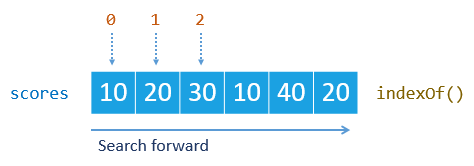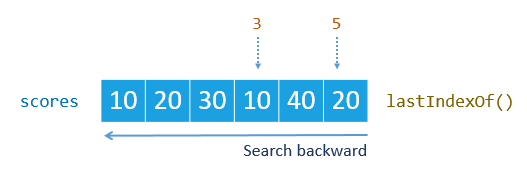### JavaScript Array – indexOf Element## JavaScript Array – indexOf Element in an ArraySummary:  how to use the JavaScript array `indexOf()` and `lastIndexOf()` methods to find the position of an element in an array.

## Introduction to the JavaScript array `indexOf()` method

To find the position of an element in an array, use the `indexOf()` method. This method returns the index of the first occurrence the element, or -1 if the element is not found.

The following illustrates the syntax of the `indexOf()` method.

```Array.indexOf(searchElement, fromIndex) ```
`Code language: JavaScript (javascript)`

As shown above, the `indexOf()` method accepts two named arguments.

1. The `searchElement `argument is the element that you want to find in the array.
2. The `fromIndex` is an array index at which the function starts the search.

The `fromIndex` argument can be a positive or negative integer. If the `fromIndex` argument is negative, the `indexOf()` method starts searching at array’s length plus `fromIndex`.

In case omit the `fromIndex` argument, the `indexOf()` method starts searching from the begining of the string.

Notice that the `indexOf()` method uses the strict equality comparison algorithm that is similar to the triple-equals operator (`===`) when comparing the `searchElement` with the elements in the array.

## The JavaScript array `indexOf()` method examples

Suppose, an array `scores` that consists of six numbers as follows:

`var scores = [10, 20, 30, 10, 40, 20];`
`Code language: JavaScript (javascript)`

The following example uses the `indexOf()` method to find the elements in the `scores` array:

```console.log(scores.indexOf(10)); // 0 console.log(scores.indexOf(30)); // 2 console.log(scores.indexOf(50)); // -1 console.log(scores.indexOf(20)); // 1```
`Code language: JavaScript (javascript)`And the following example uses the `fromIndex()` with the negative values:

```console.log(scores.indexOf(20,-1)); // 5 (fromIndex = 6+ (-1) = 5) console.log(scores.indexOf(20,-5)); // 1 (fromIndex = 6+ (-5) = 1)```
`Code language: JavaScript (javascript)`

Assuming that the following array of objects, where each object has two properties: `name` and `age`.

```var guests = [ {name: 'John Doe', age: 30}, {name: 'Lily Bush', age: 20}, {name: 'William Gate', age: 25} ];```
`Code language: JavaScript (javascript)`

The following statement returns `-1` even though the first element of the `guests` array and the `searchElement` have the same values in the `name` and `ages` properties. This is because they are two different objects.

```console.log(guests.indexOf({ name: 'John Doe', age: 30 })); // -1```
`Code language: JavaScript (javascript)`

Sometimes, you want to find the indices of all occurrences of an element in an array. The following `find()` function uses the `indexOf()` method to do so.

```function find(needle, haystack) { var results = []; var idx = haystack.indexOf(needle); while (idx != -1) { results.push(idx); idx = haystack.indexOf(needle, idx + 1); } return results; }```
`Code language: JavaScript (javascript)`

The following example uses the `find()` function above to return an array of positions of the number 10 in the `scores` array.

`console.log(find(10,scores)); // [0, 3]`
`Code language: JavaScript (javascript)`

## JavaScript array `lastIndexOf()` method

The Array type has another method called `lastIndexOf()` that provides the similar functionality to the `indexOf()` method.

The following illustrates the syntax of the `lastIndexOf()` method:

`Array.lastIndexOf(searchElement[, fromIndex = Array.length - 1])`
`Code language: JavaScript (javascript)`

The `lastIndexOf()` method returns the index of the last occurrence of the `searchElement` in the array. It returns `-1` if it cannot find the element.

Different from the `indexOf()` method, the `lastIndexOf()` method searches for the element backward, starting at `fromIndex.`

The following statements return the last indices of the number 10 and 20 in the `scores` array.

```console.log(scores.lastIndexOf(10));// 3 console.log(scores.lastIndexOf(20));// 5 ```
`Code language: JavaScript (javascript)`Because the number 50 is not in the `scores` array, the following statement returns `-1`.

`console.log(scores.lastIndexOf(50));// -1`
`Code language: JavaScript (javascript)`

In this tutorial, you have learned how to use the JavaScript array `indexOf()` and `lastIndexOf()` methods to locate an element in the array.